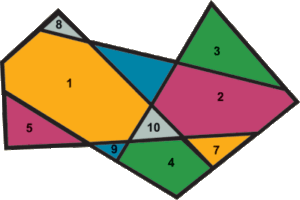# Inner-cover of Non-convex Shapes

## Abstract

We present an algorithm that for a given simple non-convex polygon P finds an approximate inner-cover by large convex polygons. The algorithm is based on an initial partitioning of P into aset C of disjoint convex polygons which are an exact tessellation of P. The algorithm then builds a set of large convex polygons contained in P by constructing the convex hulls of subsets of C. We discuss different strategies for selecting the subsets and we claim that in most cases our algorithm produces an effective approximation of P.

We implemented the inner-cover algorithm presented in this paper and developed an interactive application to test it. The user can set the coverage ratio and the limit on the number of polygons, as well as choosing the cover strategy and face order. Our implementation was developed using the CGAL library of geometric algorithm. In particular we used the planar maps and arrangements package.

• Daniel Cohen-Or, Shuly Lev-Yehudi, Adi Karol, and Ayellet Tal
Inner-cover of Non-convex Shapes
The 4th Israel-Korea Bi-National Conference on Geometric Modeling, Tel-Aviv, 2002

Shuly Lev-Yehudi